Function Repository Resource:

# StrictIntegerCompositions

Generate the zero-free compositions of a positive integer

Contributed by: Wolfram Staff
 ResourceFunction["StrictIntegerCompositions"][n] gives a list of all possible ways to form a composition of the integer n into smaller integers. ResourceFunction["StrictIntegerCompositions"][n,k] gives compositions using at most k integers. ResourceFunction["StrictIntegerCompositions"][n,{k}] gives compositions into exactly k integers. ResourceFunction["StrictIntegerCompositions"][n,{kmin,kmax}] gives compositions into between kmin and kmax integers. ResourceFunction["StrictIntegerCompositions"][n,kspec,{s1,s2,…}] gives compositions involving only the numbers si. ResourceFunction["StrictIntegerCompositions"][n,kspec,sspec,m] limits the result to using only first m partitions.

## Details and Options

ResourceFunction["StrictIntegerCompositions"] includes all possible permutations of compositions given by IntegerPartitions.
ResourceFunction["StrictIntegerCompositions"][n] is equivalent to ResourceFunction["StrictIntegerCompositions"][n,All].
ResourceFunction["StrictIntegerCompositions"][n,{kmin,kmax,dk}] gives compositions into kmin, kmin+dk, … integers.
n and the si can be rational numbers, and can be negative.
In the list of compositions, those involving earlier si are given last.
In ResourceFunction["StrictIntegerCompositions"][n,kspec,sspec,m], a kspec of All corresponds to {0,Infinity}, an sspec of All corresponds to Range[n] and an m of All corresponds to Infinity.

## Examples

### Basic Examples (1)

All the strict compositions of an integer:

 In:=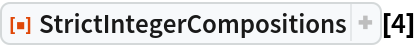Out=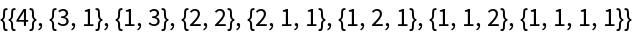### Scope (6)

All the strict compositions of 5:

 In:=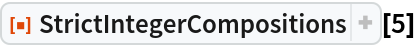Out=The compositions of 5 into at most three integers:

 In:=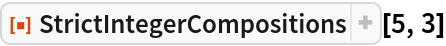Out=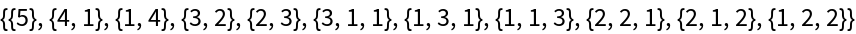Compositions that involve only 1, 2 and 4:

 In:=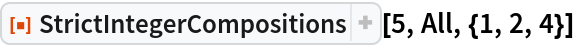Out=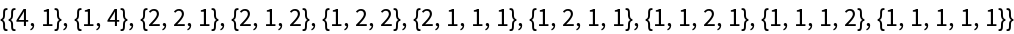Compositions of even length only:

 In:=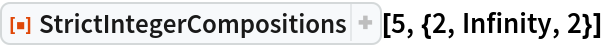Out=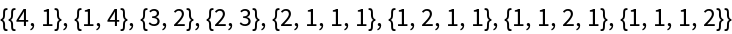Ways to form 3 from any of five given rational numbers:

 In:=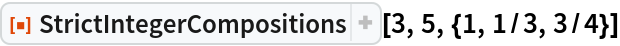Out=Limit the number of results:

 In:=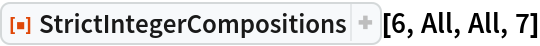Out=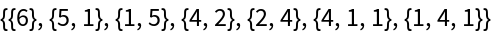### Properties and Relations (5)

There are 2n-1 strict compositions of n:

 In:=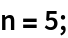In:=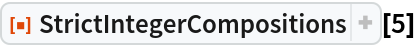Out=In:=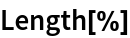Out=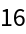In:=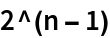Out=Each composition adds up to the original number:

 In:=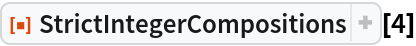Out=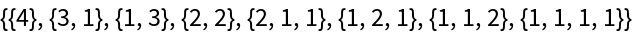In:=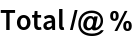Out=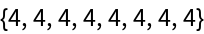StrictIntegerCompositions gives the permutations of compositions returned by IntegerPartitions:

 In:=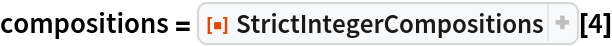Out=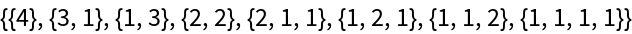In:=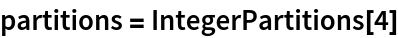Out=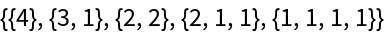In:=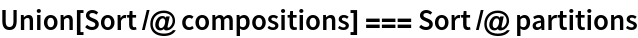Out=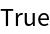Results of StrictIntegerCompositions do not include zeros, whereas results of the resource function IntegerCompositions do:

 In:=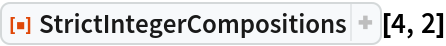Out=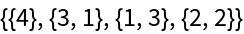In:=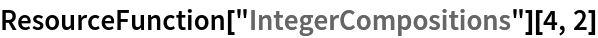Out=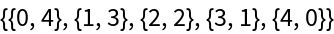Unlike the resource function IntegerCompositions, StrictIntegerCompositions does not necessarily give compositions in canonical order:

 In:=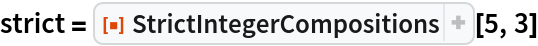Out=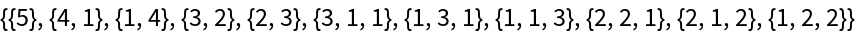In:=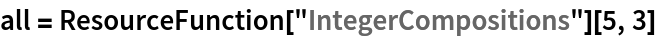Out=In:=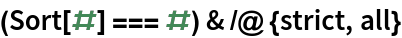Out=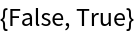### Possible Issues (3)

StrictIntegerCompositions cannot give an infinite list of compositions:

 In:=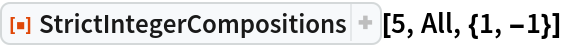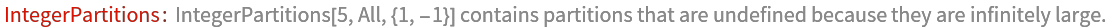Out=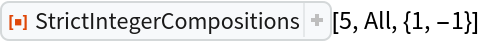In:=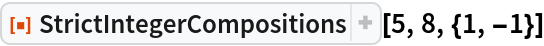Out=There are no strict compositions of 1/2:

 In:=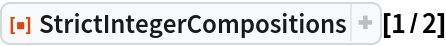Out=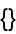There are, however, compositions into rationals:

 In:=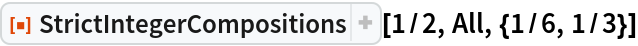Out=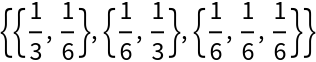If all items requested by the fourth argument are not present, as many as possible are returned:

 In:=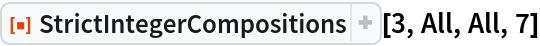Out=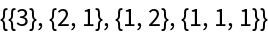### Neat Examples (1)

A compact way to show integer compositions:

 In:=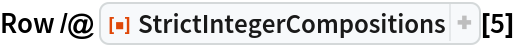Out=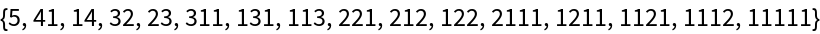## Version History

• 1.0.0 – 06 March 2020